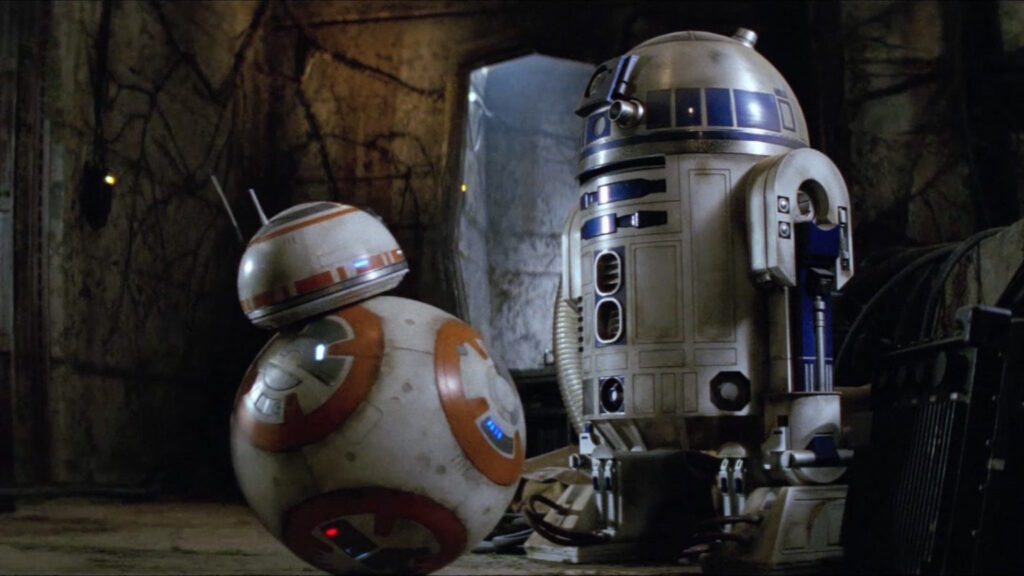In this post, we’ve partnered with Teach Kids Robotics to help explain how robots work. We’ve divided the question into separate lessons, each covering a specific topic in robotics that answers how do robots work. Feel free to skip to the lesson you are most interested in, but it is recommended to read through these lessons in order. After reading through all of these lessons, you will know how robots work. So, how do robots work?Lesson 1: What is a robot?

Summary: In this post, we’ll cover what a robot is, how it is defined, the key components that make up a robot and provide some example real robots in the world and movies. So, what is a robot?

Key Topics Explained: Sense-Plan-Act

Lesson 2: How do robots experience the world?

Summary: In this post, we’ll cover how robots experience the world, first understanding how us humans experience the world, and then drawing parallels to robots with examples of how a robot experiences the world. So, how do robots experience the world?

Key Topics Explained: Sensors

Lesson 3: How do robots see?

Summary: In this post, we’ll cover how robots see, focusing on two of the most common kinds of sensors in robotics: cameras and LiDARs. We also provide image examples that help explain how a robot translates what its sensors see to data. So, how do robots see?

Key Topics Explained: Cameras, Computer Vision, LiDARs, Point Clouds

Lesson 4: How do robots know where they are?

Summary: In this post, we’ll cover how a robot knows where it is which allows it to move around its environment and specifically to a goal destination. Additionally we provide numerous image representations of how a robot represents the world and navigates with in it. So, how do robots know where they are?

Key Topics Explained: Mapping, Occupancy Grids, Localization, Pose, Coordinate Frames, SLAM, Particle Filtering, Kalman Filter

Lesson 5: How does a robot decide what to do?

Summary: In this post, we’ll cover what determines a robots actions. Why do robots behave or move they way they do? What is the goal of a given robot? We explain these questions with numerous illustrations to highlight robot decision making. So, how does a robot decide what to do?

Key Topics Explained: Planning, Path Planning, Cost function, Heuristic, Costmap, Control Loop, Closed Loop, Open Loop

We hope after reading through the above lessons, you can now explain to others how robots work, and no longer wonder how do robots work?